Next: Bi-directional Estimators for Light Up: A Review of Monte Previous: Monte Carlo Light Tracing

# Bi-directional Path Tracing

In this section a hybrid two-pass algorithm is introduced that has been proposed by Lafortune which does not need mesh generation either [LW93]. This method is based on the bi-directional particle tracing since particles are shot at the same time from a selected light source and the viewpoint. After this, shadow rays are used to connect each intersection point in the light path with each intersection point in the eye path, and contributions are added to the flux of the relevant pixel.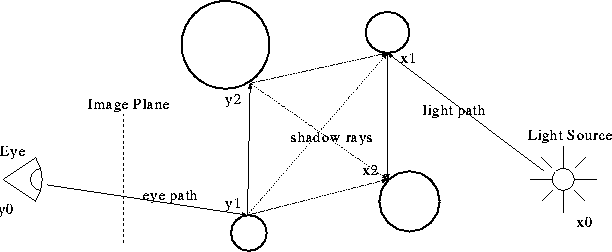Figure: Bi-directional path tracing

Random walks can be written as:

•for the light path, where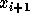is the point seen by pointalong direction, and
•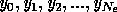for the eye path, where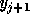is the point that sees pointalong direction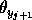.

The direction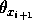in the light path is sampled according to the subcritical pdf (spdf):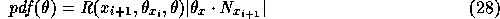where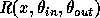is the bi-directional reflection distribution function (BRDF). The directionin the eye path is sampled the same way:After generating a light path and an eye path all intersection points are linked by shadow rays. The flux is estimated by a weighted sum:where factors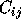express the estimates of the flux found by i reflections on the eye path and j reflections on the eye path. These factors are evaluated differently according to the following three cases:

•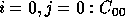This term is an estimate for the flux received from a light source that is directly seen through the pixel under consideration.
•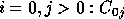This term is an estimate for the flux that reaches the eye from the light source through the eye path, as in classical path tracing.
•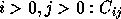This term is an estimate for the flux that reaches the eye from the light source, trough i reflections on the light path and j reflections on the eye path.

There are various alternatives to choose the weights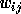for the contributions. For example the classical path tracing is the special case of this generic algorithm using the following weights: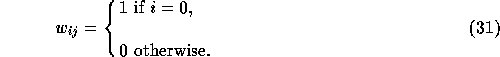Next: Bi-directional Estimators for Light Up: A Review of Monte Previous: Monte Carlo Light Tracing

Csébfalvi Balázs
Tue Apr 15 18:39:13 METDST 1997